# Introduction to circacompare

## Updates since the initial release (circacompare 0.1.0) and Bioinformatics journal article.

There have been several added features to the current version of the circacompare package since the publication in Bioinformatics and initial release on CRAN. Many of the possible uses for this package are not described in the manuscript. The approach to compare two groups of rhythmic data, regarding mesor, amplitude and phase, as described in the manuscript, can be completed by using the circacompare() function under the default settings in any version of the package. In addition to this, the current version offers approaches to:

• Perform analysis on a single rhythmic dataset to estimate its mesor, amplitude and phase.
• Choose to use a known period (user-determined) or to let the model estimate the period from the data.
• Add parameters to estimate the exponential decay in any of the rhythmic characteristics.
• Use a mixed-model instead of a fixed effects model to take into account within-subject correlation regarding any set of rhythmic characteristics.
• Perform a comparison between groups regarding all or a subset of rhythmic characteristics.

## Introduction to circacompare, using fixed-effects only models and no customized model parametrization

### Input data

The first part of using circacompare to analyse your data is to ensure that your data is formatted correctly. All of the functions within the circacompare package expect that your data will be of a tidy format, meaning that each row will contain only one observation, with columns to represent the time, group or subject ID for that observation.

In the simplest case, you may have a single rhythm for which you’re wanting to estimate the mesor, amplitude and phase. In this case, you only need a variable for the time of observation and the outcome which you’re wanting to model.

library(circacompare)
library(ggplot2)
set.seed(42)
data_single <- make_data(k1=0, alpha1=0, phi1=0, noise_sd=1)[c("time", "measure")]
#>   time    measure
#> 1    1 11.0302167
#> 2    2  8.0955559
#> 3    3  7.4341962
#> 4    4  5.6328626
#> 5    5  2.9924588
#> 6    6 -0.1061245

In the case that you have data from two groups and you’re wishing to determine the differences in mesor, amplitude, or phase between them, you will need an additional column (with two possible values) representing the groups.

set.seed(42)
data_grouped <- make_data(phi1=6, noise_sd=1)
#>   time    measure group
#> 1    1 11.0302167    g1
#> 2    2  8.0955559    g1
#> 3    3  7.4341962    g1
#> 4    4  5.6328626    g1
#> 5    5  2.9924588    g1
#> 6    6 -0.1061245    g1
tail(data_grouped)
#>    time  measure group
#> 91   43 11.64979    g2
#> 92   44 12.63275    g2
#> 93   45 15.92162    g2
#> 94   46 17.98846    g2
#> 95   47 15.88601    g2
#> 96   48 15.58159    g2

### circa_single()

circa_single() is used to analyse a single rhythm and provide estimates of its mesor, amplitude and phase.

result <- circa_single(x=data_single, col_time="time", col_outcome="measure", period=24)

result
#> $fit #> Nonlinear regression model #> model: measure ~ k + (alpha) * cos((1/period) * time_r - (phi)) #> data: x #> k alpha phi #> 0.05322 10.07049 0.01645 #> residual sum-of-squares: 101.8 #> #> Number of iterations to convergence: 4 #> Achieved convergence tolerance: 1.746e-06 #> #>$summary
#>         parameter        value
#> 1      rhythmic_p 2.524753e-80
#> 2           mesor 5.322134e-02
#> 3       amplitude 1.007049e+01
#> 5 peak_time_hours 6.283545e-02
#> 6          period 2.400000e+01
#>
#> $plot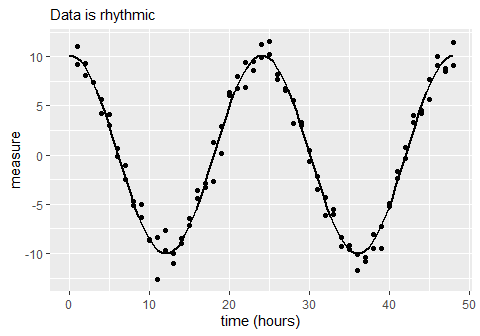The fitted model is included as the first element of the results. It fits a model: measure ~ k + alpha * cos(time_r - phi) where • measure is the outcome of interest • k is the mesor • alpha is the amplitude • time_r is the time in radian-hours, and • phi is the amount of phase shift (from time=0) in radian-hours. The parameter estimates of time in radian-hours (time_r and phi) are converted back to hours and reported in the data.frame (second element of list) and x-axis of the graph (third item of list) ### circacompare() circacompare() is used to analyse a dataset with two groups of rhythmic data. It fits a model to estimate and statistically support differences in mesor, amplitude and phase between the two groups. result2 <- circacompare(x=data_grouped, col_time="time", col_group="group", col_outcome="measure") result2 #>$plot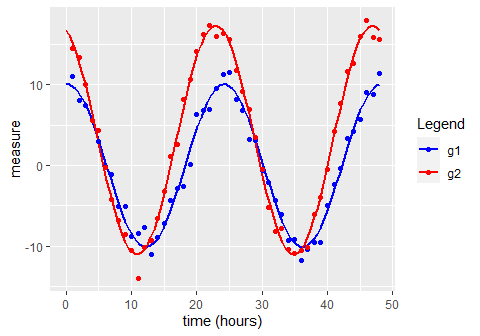#>
#> $summary #> parameter value #> 1 Presence of rhythmicity (p-value) for g1 1.743971e-38 #> 2 Presence of rhythmicity (p-value) for g2 2.669822e-49 #> 3 g1 mesor estimate -4.182883e-02 #> 4 g2 mesor estimate 3.148272e+00 #> 5 Mesor difference estimate 3.190100e+00 #> 6 P-value for mesor difference 1.012031e-26 #> 7 g1 amplitude estimate 1.006017e+01 #> 8 g2 amplitude estimate 1.414767e+01 #> 9 Amplitude difference estimate 4.087507e+00 #> 10 P-value for amplitude difference 5.276837e-24 #> 11 g1 peak time hours 2.044428e-01 #> 12 g2 peak time hours 2.287166e+01 #> 13 Phase difference estimate -1.332787e+00 #> 14 P-value for difference in phase 9.919731e-24 #> 15 Shared period estimate 2.400000e+01 #> #>$fit
#> Nonlinear regression model
#>   model: measure ~ (k + k1 * x_group) + ((alpha + alpha1 * x_group)) *     cos((1/period) * time_r - ((phi + phi1 * x_group)))
#>    data: x
#>        k       k1    alpha   alpha1      phi     phi1
#> -0.04183  3.19010 10.06017  4.08751  0.05352 -0.34892
#>  residual sum-of-squares: 94.25
#>
#> Number of iterations to convergence: 4
#> Achieved convergence tolerance: 1.485e-07

This fits a model: measure ~ k + k1 * x_group + (alpha + alpha1 * x_group) * cos(time_r - (phi + phi1 * x_group)) where

• x_group is a dummy variable which represents the different groups: x_group=0 and x_group=1 for the first and second group, respectively

• measure is the outcome of interest

• k is the mesor of the first group

• k1 is the difference in mesor between the first and second group

• alpha is the amplitude of the first group

• alpha1 is the difference in amplitude between the first and second group

• time_r is the time in radian-hours

• phi is the amount of phase-shift of the first group (from time=0) in radian-hours, and

• phi1 is the amount of phase-shift of the second group from the first group in radian-hours

The time-related parameter estimates (phi and phi1) are converted from radian-hours to hours before being used to report g1 peak time, g2 peak time, and phase difference estimate.

The second item of the result2 list is a data.frame containing the important results from the model. It returns estimates and for the rhythmic parameters for each group as well as the p-values associated with those which represent differences between the groups (k1, alpha1, phi1).

More detailed outputs from the model can be obtained from the model itself

### When to use what

If you are looking to estimate the rhythmic parameters of a single group, use circa_single(). If you are looking to estimate the differences between two rhythmic datasets, use circacompare()

If your data has a hierarchical structure, a mixed model may be more appropriate (keep reading). This may be the case if you have repeated measurements from the same subjects/tissues over time, for example. In this case, consider the equivalents of the above: circa_single_mixed() and circacompare_mixed(). In addition to what has been described, these mixed models require the user to specify which parameters ought to have a random effect and the identifying column (col_id) for this hierarchical structure.

## Mixed-model variants and how to customize the parameterization of the model

### Firstly, a mention on control and model parameters

When we refer to parameters, we are talking about covariates in the model being fit that will represent rhythmic characteristics of the data. Arguments are what the user (you) supplies when calling the function.

For all of the circacompare functions, the argument control contains a list of optional arguments that can be used to modify the parameterization of the model being fit. This list includes main_params, decay_params, rand_params, group_params which are all character vectors. The naming conventions of values within these vectors is consistent with our model formulas described earlier: “k” for mesor, “alpha” for amplitude, “phi” for phase. The additional parameter we introduce here is “tau” for period.

In circa_single() there are no groups to model differences between so group_params is ignored. Since there are also no random effects, rand_params is also ignored. Only the main_params and decay_params are considered. Similarly, circacompare() considers main_params, decay_params, and ignores rand_params(), but also includes group_params as it is used to consider the differences between groups. Only the mixed-model variants of these two functions, circa_single_mixed() and circacompare_mixed(), consider rand_params.

For the main_params vector, the possible values are k for the mesor, alpha for amplitude, phi for phase, and tau. The only optional parameter of these in the main_params is tau, the rest must be included at all times. The default is main_params=c("k", "alpha", "phi"), which excludes the parameterization of period in the model.

The values within decay_params must be a set within main_params as, for example, we can’t model the decay in period, without modeling the starting period. The default is to not model decay of any rhythmic characteristics: decay_params=c().

Additional possible arguments in the control list are:

• period_param adds "tau" to the main_params if TRUE; default: period_param=FALSE.
• period_min estimated bottom range for period starting values when performing nonlinear regression; default: period_min=20.
• period_max estimated upper range for period starting values when performing nonlinear regression; period_max=28,

If you are modeling non-circadian (but still rhythmic) data, with a much larger or smaller period than 24 hours, and you want to have the model estimate the period (tau) you can change period_min and period_max to more realistic ranges. This may reduce the time required for the model to converge.

Note that when adding parameters to decay_params, you should call the name of the parameter in as it is in main_params - decay_params=c("k"), for example. This will add a new parameter k_decay to the model. If you want to add a grouping parameter to this decay parameter, you need to call the decay of mesor explicitly, group_params=c("k_decay"), as "k" alone would be referring to the mesor value, not the decay of mesor over time.

Similarly, when adding parameters as random-effects by adding to rand_params, we need to call the parameter name (including it’s suffix: “_decay” for decay parameters and “1” for group parameters, or “_decay1” for grouped decay parameters). If I wanted to fit a model with the default main_params as well as period (tau), a decay in mesor (k), and grouping on mesor decay rate, amplitude and phase, but not starting mesor:

control=list(main_params=c("k", "alpha", "phi", "tau"), decay_params=c("k"), group_params=c("k_decay", "alpha", "phi"))

The resulting model will assume that both groups have a shared mesor and period as "k" and "tau", respectively, are excluded from group_params. The model will estimate the period from the data. It will model the exponential decay in mesor as well as the influence of group assignment on this rate of decay.

### Examples

If you have repeated measures data, then it may be inappropriate to use a standard fixed effects only model. In this case, you should use a mixed model, with context-relevant random effects for the ID/subjects/mice from which you’re obtaining repeated observations.

Here, I have some data that has some simulated within-id correlation in terms of the amount of phase shift between groups.

set.seed(42)
phi1_in <- 3
mixed_data <- function(n){
counter <- 1
for(i in 1:n){
x <- make_data(k1=0, alpha1=5, phi1=rnorm(1, phi1_in, 1), hours=72, noise_sd = 2)
x$id <- counter counter <- counter + 1 if(i==1){res <- x}else{res <- rbind(res, x)} } return(res) } df <- mixed_data(20) out <- circacompare_mixed( x = df, col_time = "time", col_group = "group", col_outcome = "measure", col_id = "id", control=list(grouped_params=c("alpha", "phi"), random_params=c("phi1")), period=24 ) ggplot(data=df[df$id %in% c(1:6),], aes(time, measure)) +
geom_point(aes(col=group)) +
geom_smooth(aes(group=interaction(as.factor(id), group)), span=0.3)+
facet_wrap(~id)
#> geom_smooth() using method = 'loess' and formula 'y ~ x'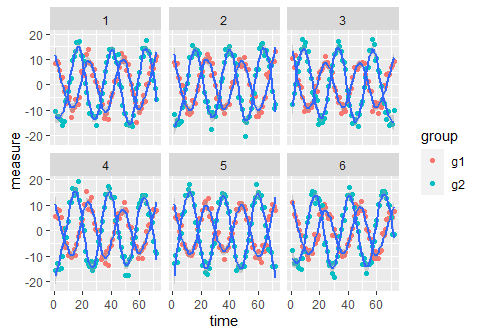For each subject (id), there are measurements in both groups (g1 and g2) but the degree of change in phase between g1 to g2 is subject-dependent. The phase of group 1 is not subject-dependent, though, as each subject has approximately the same phase in g1. However, it’s the amount of phase shift from g1 to g2 that is subject-dependent. This is equivalent to each subject having a random slope term but not a random intercept. In an experimental context, this could be the case when mice are kept under light-dark (LD) conditions g1 and then moved to constant conditions (LL or DD), g2. We would expect the phase to be consistent under the same conditions in g1 but the change in phase when they are moved to LL or DD could be mouse-dependent.

In this scenario, we want to have a “random-slope” on the phase, so we should include phi1 as a random effect. Also, since we know (from the data generating process) that both groups share the same mesor but not amplitude, we included alpha and phi as grouped parameters, but excluded k. We also know that the period is 24 hours, so we didn’t bother fitting a parameter period either (adding "tau" to main_params) so we left main_params at its default value: main_params=c("k", "alpha", "phi"). In the code above, we used: control=list(grouped_params=c("alpha", "phi"), random_params=c("phi1"))

From the data generating process used above, we expect:

• phi1=3
• alpha1=5
out$plot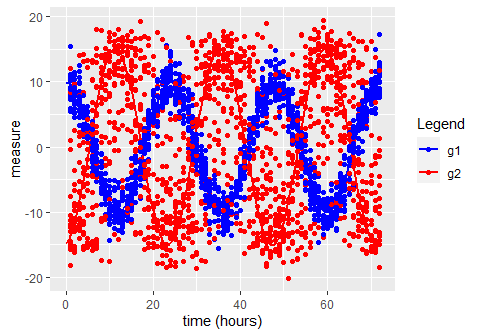summary <- as.data.frame(circacompare:::extract_model_coefs(out$fit))
summary$95% CI ll <- summary$estimate - summary$std_error*1.96 summary$95% CI ul <- summary$estimate + summary$std_error*1.96

summary
#>             estimate   std_error      p_value   95% CI ll   95% CI ul
#> k      -0.0119537550 0.037332883 7.488449e-01 -0.08512621  0.06121870
#> alpha   9.9620621275 0.074665639 0.000000e+00  9.81571747 10.10840678
#> phi    -0.0008642419 0.007494939 9.082075e-01 -0.01555432  0.01382584
#> alpha1  5.0858216997 0.105593161 0.000000e+00  4.87885910  5.29278430
#> phi1    2.9628387009 0.265371385 2.320667e-28  2.44271079  3.48296662

The effects were all pretty well estimated, given the 95% confidence intervals from the model outputs. If we expect the subject’s to have different starting phases but their change in phase between groups to be the same, we may have put the random effect on phi rather than phi1.

Perhaps more commonly applicable would be if each subject has some random mesor or baseline expression level. In this case, it may be worthwhile to include k as a random effect. If k is included, this is equivalent to giving all subjects a “random intercept” as baseline levels of the outcome for each subject will be the random effect.

## Period and decay parameters

There is the option to add parameters to represent the decay of any rhythmic characteristic as well as the difference between groups for this decay. For model below, we make simulated data with no difference in mesor or phase between groups, a randomly generated period between 8 and 20 hours that we want to estimate (and will check at the end). We also have one group which has exponentially decaying amplitude at the rate:

$\alpha*e^{-\alpha_{decay}*t}$ Where alpha is the amplitude, and t is time in radian-hours.

In our case, alpha_decay will be another randomly generated value, somewhere between 0.02 and 0.05, that we will check at the end.

To model these data, we will include all the standard parameters as well as tau (to estimate the period) in the main_params: main_params=c("k", "alpha", "phi", "tau")

We will include the term for amplitude (alpha) in the decay_params: decay_params=c("alpha")

And we will model the differences between groups for both the starting amplitude,alpha, and its decay over time alpha_decay: grouped_params=c("alpha", "alpha_decay")

This produces the following model:

measure~k+((alpha+alpha1*x_group)*exp(-(alpha_decay+alpha_decay1*x_group)*time_r))*cos((24/(tau))*time_r-(phi))

set.seed(42)
tau_in <- runif(1, 8, 20)
alpha_decay1_in <- runif(1, 0.02, 0.05)

df <- make_data(k1=0, alpha1=10, phi1=0, seed=42, hours=120, noise_sd=2)
df$time <- df$time/24*tau_in

# Note that when decay is estimated, it is on a scale of time in hours. There is no relation decay rate and the period.
df$measure[df$group=="g2"] <- df$measure[df$group=="g2"]*exp(-alpha_decay1_in*df$time[df$group=="g2"])

out_alpha_decay <-
circacompare(x=df, "time", "group", "measure", period=NA,
control=list(
main_params=c("k", "alpha", "phi", "tau"),
decay_params=c("alpha"),
grouped_params=c("alpha", "alpha_decay")
))

out_alpha_decay$plot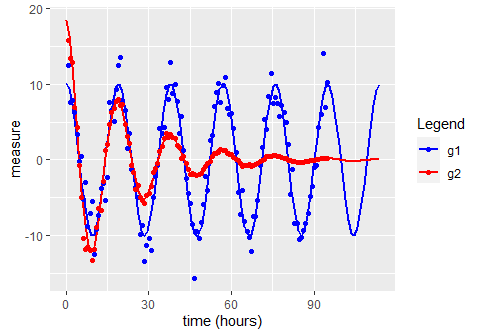summary <- as.data.frame(circacompare:::extract_model_coefs(out_alpha_decay$fit))
summary$95% CI ll <- summary$estimate - summary$std_error*1.96 summary$95% CI ul <- summary$estimate + summary$std_error*1.96
summary\$p_value <- NULL
summary
#>                   estimate    std_error    95% CI ll    95% CI ul
#> k            -3.215705e-02 0.1004811657 -0.229100134  0.164786035
#> alpha         1.009538e+01 0.4013321243  9.308773100 10.881995027
#> alpha_decay   5.929789e-05 0.0007177633 -0.001347518  0.001466114
#> alpha1        8.370390e+00 0.9428234990  6.522455970 10.218324086
#> alpha_decay1  4.457242e-02 0.0029372104  0.038815485  0.050329349
#> tau           1.896892e+01 0.0339990036 18.902277678 19.035553773
#> phi           2.045861e-02 0.0281330329 -0.034682136  0.075599353

We now have estimates and 95% confidence intervals for the difference in amplitude decay between groups (alpha_decay1) and the period (tau) that were of interest. Lets see the values we used to generate the data and check that the confidence intervals were appropriate.

cat("Real period: ", tau_in, "\n",
"Real alpha_decay: ", alpha_decay1_in, sep="")
#> Real period: 18.97767
#> Real alpha_decay: 0.04811226

Looks like our estimates and confidence intervals suited our now-known data generating process well!

## Limitations

### Decay functions

Currently, decay parameters are used to model exponential decay as this seemed sensible for most biological contexts. However, users may want to model decay as a linear (or some other) function. There is no implementation for this in the current package. If this is of interest to you, please contact me or create an issue on GitHub.

### Period and phase parameters

It may be tempting to investigate the differences between two groups of rhythmic data regarding everything! But, unfortunately, there are limitations. If two groups of data are allowed to vary regarding their period, the interpretation of a difference in phase is no longer appropriate. For example, if two rhythms have different periods, at what time are you wanting to know the difference in phase between the groups? The amount of phase-shift will differ over time if they have different periods. For this reason, you cannot have both tau and phi within group_params in either circacompare() or circacompare_mixed().

### Model diagnostics and choice

When using circa_single() or circacompare() a nonlinear regression model (with fixed-effects only) of class nls is fit. When using circa_single_mixed() or circacompare_mixed() a nonlinear mixed regression model of class nlme is fit. Users should be aware of the assumptions of these models when interpreting the results. See here for a thorough discussion of model assumptions and performing diagnostic tests using the {nlstools} package.